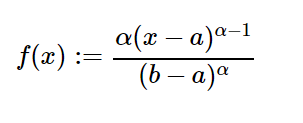Related Articles
sympy.stats.PowerFunction() in Python
• Last Updated : 08 Jun, 2020

With the help of `sympy.stats.PowerFunction()` method, we can get the continuous random variable which represents the Power Function distribution.Syntax : `sympy.stats.PowerFunction(name, alpha, a, b)`
Where, a, b and alpha are real number.

Return : Return the continuous random variable.

Example #1 :
In this example we can see that by using `sympy.stats.PowerFunction()` method, we are able to get the continuous random variable representing power function distribution by using this method.

 `# Import sympy and PowerFunction ` `from` `sympy.stats ``import` `PowerFunction, density ` `from` `sympy ``import` `Symbol, pprint ` ` `  `z ``=` `Symbol(``"z"``) ` `alpha ``=` `Symbol(``"alpha"``, positive ``=` `True``) ` `a ``=` `Symbol(``"a"``, positive ``=` `True``) ` `b ``=` `Symbol(``"b"``, positive ``=` `True``) ` ` `  `# Using sympy.stats.PowerFunction() method ` `X ``=` `PowerFunction(``"x"``, alpha, a, b) ` `gfg ``=` `density(X)(z) ` ` `  `print``(gfg) `

Output :

(-2*a + 2*z)/(-a + b)**2

Example #2 :

 `# Import sympy and PowerFunction ` `from` `sympy.stats ``import` `PowerFunction, density, variance ` `from` `sympy ``import` `Symbol, pprint ` ` `  `z ``=` `Symbol(``"z"``) ` `alpha ``=` `2` `a ``=` `0` `b ``=` `1` ` `  `# Using sympy.stats.PowerFunction() method ` `X ``=` `PowerFunction(``"x"``, alpha, a, b) ` `gfg ``=` `density(X)(z) ` ` `  `pprint(variance(gfg)) `

Output :

1/18

Attention geek! Strengthen your foundations with the Python Programming Foundation Course and learn the basics.

To begin with, your interview preparations Enhance your Data Structures concepts with the Python DS Course.

My Personal Notes arrow_drop_up
Recommended Articles
Page :